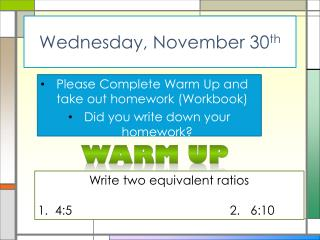DownloadDownload PresentationWednesday, November 30 th

# Wednesday, November 30 th

Télécharger la présentation## Wednesday, November 30 th

- - - - - - - - - - - - - - - - - - - - - - - - - - - E N D - - - - - - - - - - - - - - - - - - - - - - - - - - -
##### Presentation Transcript

1. Wednesday, November 30th Please Complete Warm Up and take out homework (Workbook) Did you write down your homework? Warm Up Write two equivalent ratios 1. 4:5 2. 6:10

2. Corny Joke of the Day Where do fish go on vacation? Fin-land

3. Attention! Next Checkpoint is FRIDAY

4. Ratio Review

5. Candy Ratios

6. Rate Review

7. The new movie “Hunger Games” is expected to draw large crowds. Several cinemas have already started making package deals for movie tickets. In your groups determine which Theater has the best deal! Mall of Georgia Regal Cinema 5 tickets for \$112 Hollywood 24 7 tickets for \$135.60

8. Homework Answers Can you find my mistakes? • 9: 12 or 3:4 • 8:16 or 1:2 • 10: 9 • 10:12 or 5:5 • 12:9 or 4:3 • 6:9, 12:18, or 2:3 • 12/14 or 6/7 • 15/9 or 5/3 • 15:14 • 9/12 or ¾

9. The Word Problems….

10. Proportions

11. Can you finish the statement? • Basketball is to hoop as soccer ball to ____________. • pumpkin is to Halloween as fireworks are to ________________ • Fish is to water as bird is to _________

12. These are all PROPORTIONS!

13. 4 2 2 6 4 2 4 8 __ __ __ __ __ __ __ __ = = = 4 1 2 1 1 2 2 3 A proportion is an equation that shows two equivalent ratios. Reading Math Read the proportion = as “two is to one as four is to two.”

14. 4 __ 8 number of hearts number of stars Modeling Proportions Write a proportion for the model. First write the ratio of hearts to stars. = Next separate the hearts and stars into two equal groups.

15. 2 4 2 __ __ __ 4 8 4 number of hearts number of stars Continued Now write the ratio of hearts to stars in each group. = A proportion shown by the model is = .

16. Let’s make our own Proportion! • 6 shapes of: _________________ • 8 shapes of:__________________ Proportion:

17. = 3 9 9 2 4 3 14 2 __ __ __ __ __ __ __ ___ = = = 15 6 5 1 2 7 4 8 6 •3 = 9 • 2 7 •2 = 14 • 1 8 • 2 = 4 • 4 5 • 9 = 3 • 15 16= 16 18 = 18 14 = 14 45= 45 This is how you prove proportions!

18. Question of the day How would you find the missing value to complete proportion

19. Method #1 Criss Cross and algebra

20. Steps • Cross multiply • Set up equation • Use inverse operations to solve for missing variable

21. 5 6n 90 n __ __ ___ ___ = 6 18 6 6 Criss-Cross and Solve! Find the missing value in the proportion. Find the cross products. 6 •n= The cross products are equal. 5 • 18 6n= 90 n is multiplied by 6. Divide both sides by 6 to undo the multiplication. = n = 15

22. Method #2 Use guess and check Caution: doesn’t work if the answer is a fraction!

23. Think: 6 x what number is 18 So, 5 x that number is n

24. You Try #1

25. You Try #2

26. You Try #3

27. You Try #4

28. You Try #5

29. Real World Application

30. f f 1 tbsp 1 tbsp ____ _____ _____ ____ 4 gal 6 gal 6 gal 4 gal According to the label, 1 tablespoon of plant fertilizer should be used per 6 gallons of water. How many tablespoons of fertilizer would you use for 4 gallons of water? Let f be the amount of fertilizer for 4 gallons of water. = = Write a proportion. 6 •f= 1 • 4 The cross products are equal.

31. tbsp tbsp gal gal ___ ___ ___ ___ tbsp tbsp gal gal Caution! In a proportion, the units must be in the same order in both ratios. = or =

32. 2 2 6f 4 __ __ __ ___ 3 6 6 3 Continued f is multiplied by 6. 6f= 4 Divide both sides by 6 to undo the multiplication. = Write your answer in simplest form. f = tbsp You would use tbsp of fertilizer for 4 gallons of water.

33. Word Problem Practice • Each group will be assigned one word problem • In your groups you must: • Show your proportion • Show work to show answer

34. Experiment For most people, the ratio of the length of their head to their total height is 1:7. Use proportions to test your measurements and see if they match this ratio.